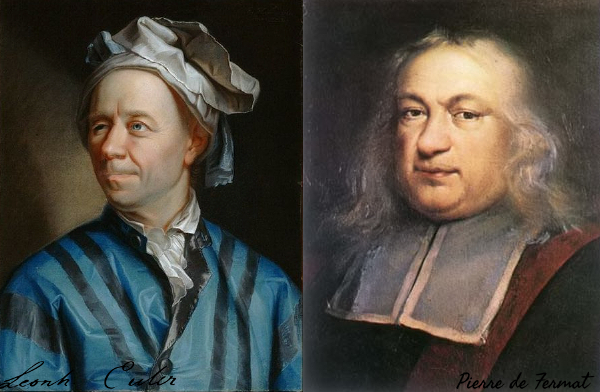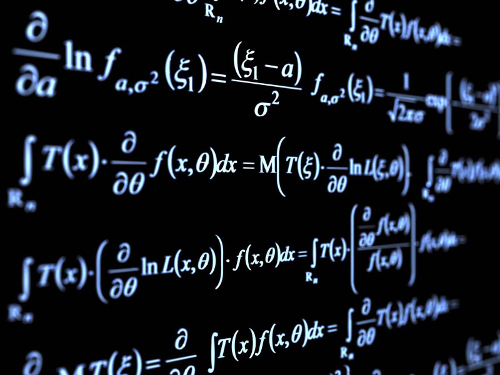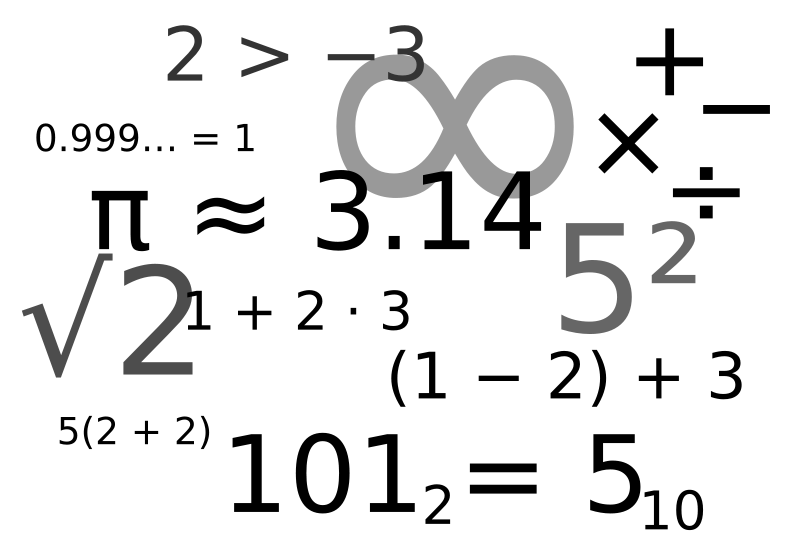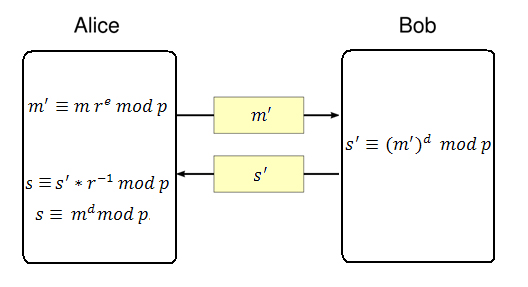## Posts

Showing posts from 2017

### Representing integers as the sum of two squaresIn this post we present a recursive algorithm for finding all the possible representations, as a sum of two squares, for any given integer that can be expressed this way.

### Representing integers as the difference of two squaresMost integers can be represented as a difference of two squares, where each square is a non-negative integer.

### Various representations for famous mathematical constantsIn this unusual post, much like in the older post,  The beauty of Infinity , we're listing the most famous mathematical constants as representations of  infinite series ,  infinite products  and limits .

### Thoughts on programming language notationsSome posts ago, we looked at what it's required in creating a new programming language . In this post we're going a little bit more into it, trying to find ways to effectively express meanings in natural ways, similar to what we can express in a natural language.

### Bacovia: a symbolic math libraryNamed after the great symbolist poet, George Bacovia , I created this library to symbolically manipulate mathematical expressions in a simple and elegant way.

### Mandelbrot setThe Mandelbrot set and its complex beauty.

### RSA algorithmRSA is a practical public-key cryptographic algorithm, which is widely used on modern computers to communicate securely over large distances. The acronym of the algorithm stands for Ron Rivest , Adi Shamir and Leonard Adleman , which first published the algorithm in 1978. # Algorithm overview Choose p and q as distinct prime numbers Compute n as n = p*q Compute \phi(n) as \phi(n) = (p-1) * (q-1) Choose e such that 1 < e < \phi(n) and e and \phi(n) are coprime Compute the value of d as d ≡ e^(-1) mod \phi(n) Public key is (e, n) Private key is (d, n) The encryption of m as c, is c ≡ m^e mod n The decryption of c as m, is m ≡ c^d mod n # Generating p and q In order to generate a public and a private key, the algorithm requires two distinct prime numbers p and q, which are randomly chosen and should have, roughly, the same number of bits. By today standards, it is recommended that each prime number to have a

### InfinitesimalsIn this post we're going to take a look at what infinitesimals are and why they are important. Infinitesimals are an abstract concept of very small values that are impossible to represent quantitatively in a finite system. # Definition We define one infinitesimal as: ε = lim_{n to \infty}\frac{1}{n} with the inequality: ε > 0. In general, the following inequalities hold true: \frac{0}{n} < \frac{1}{n} < \frac{2}{n} < ... < \frac{n}{n} as n -> \infty. # Appearance The infinitesimals appear in some fundamental limits, one of which is the limit for the natural exponentiation function: lim_{n to \infty}(1 + \frac{\x}{n})^n = \exp(\x) Using our infinitesimal notation, we can rewrite the limit as: lim_{n to \infty}(1 + ε*\x)^n = \exp(\x) where, for x=1, we have: lim_{n to \infty}(1 + ε)^n = \e. # Debate There was (and, probably, still is) a debate in mathematics whether the following limit: `lim_{n to \infty}\frac{1